#### A polygonal C-space obstacle

A simple algorithm for computing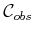exists in the case of a 2D world that contains a convex polygonal obstacle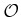and a convex polygonal robot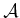. This is often called the star algorithm. For this problem,is also a convex polygon. Recall that nonconvex obstacles and robots can be modeled as the union of convex parts. The concepts discussed below can also be applied in the nonconvex case by consideringas the union of convex components, each of which corresponds to a convex component ofcolliding with a convex component of.

The method is based on sorting normals to the edges of the polygons on the basis of angles. The key observation is that every edge ofis a translated edge from eitheror. In fact, every edge fromandis used exactly once in the construction of. The only problem is to determine the ordering of these edges of. Let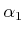,,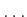,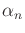denote the angles of the inward edge normals in counterclockwise order around. Let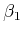,,,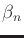denote the outward edge normals to. After sorting both sets of angles in circular order around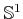,can be constructed incrementally by using the edges that correspond to the sorted normals, in the order in which they are encountered.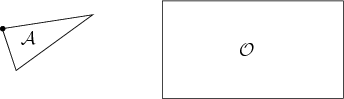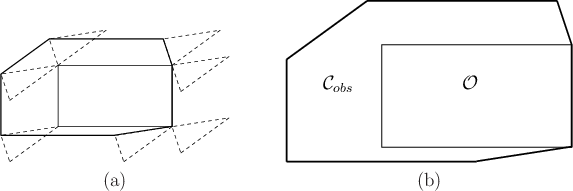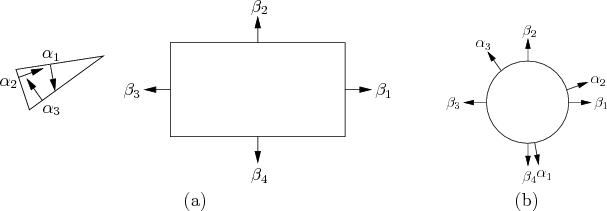Example 4..14 (A Triangular Robot and Rectangular Obstacle)   To gain an understanding of the method, consider the case of a triangular robot and a rectangular obstacle, as shown in Figure 4.13. The black dot ondenotes the origin of its body frame. Consider sliding the robot around the obstacle in such a way that they are always in contact, as shown in Figure 4.14a. This corresponds to the traversal of all of the configurations in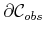(the boundary of). The origin oftraces out the edges of, as shown in Figure 4.14b. There are seven edges, and each edge corresponds to either an edge ofor an edge of. The directions of the normals are defined as shown in Figure 4.15a. When sorted as shown in Figure 4.15b, the edges ofcan be incrementally constructed.The running time of the algorithm is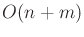, in whichis the number of edges defining, and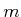is the number of edges defining. Note that the angles can be sorted in linear time because they already appear in counterclockwise order aroundand; they only need to be merged. If two edges are collinear, then they can be placed end-to-end as a single edge of.

Steven M LaValle 2020-08-14